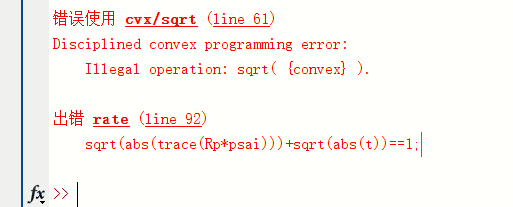# Illegal operation: sqrt( {convex} )

Hello! I was wondering if anybody can tell me how I can express the constraint sqrt(abs(trace(Rp*psai)))+sqrt(abs(t))==1 in the CVX ? The problem is that CVX declares the following error:
“Disciplined convex programming error:Illegal operation: sqrt( {convex} ).”
Thank you very much!!`sqrt(abs(t))` is neither convex nor concave, so you couldn’t even upper or lower bound it. Using it in a nonlinear equality only makes it even worse.

Thank you for your tips! But how can I solve this problem?

Since your problem is nonconvex cvx cannot be used.

You can try the nonconvex optimizer in yalmip.